Mobile QR Code1. (Department of Electronics and Electrical Engineering, Dankook University, Yong-In, Korea)

Sorting grid, sorting engine, sorting module, compare free sorting, asynchronous sorting unit

## I. INTRODUCTION

Sorting is a widely used prime function in most of the data-centric applications such as big data , image processing , database query operations , wireless local area network , ATM switching , etc. Due to the great impact of sorting operations, a lot of software algorithms and hardware implementations have been developed. In many cases, especially for real-time sorting operations [6,7], software-oriented designs are difficult to meet timing constraints. Since hardware implementation has many advantages over software approaches, developing an efficient hardware sorting unit has been a significant challenge to overcome the computational bottleneck of the sorting problems.

Most prior works on hardware sorting designs are implemented based on some modification of traditional sort algorithms or some altered structure of switching network with parallel processing and pipelining stages. Most of these approaches are based on compare-and-swap (CAS) operation which compares the magnitudes of two inputs and switches their positions according to the result. To improve the performance of sorting architectures, parallel data-independent CAS blocks are devised to form sorting network [8-10]. The CAS based sorting architectures recursively move inputs from memory to memory so that they require large memory space and processing time for moving inputs.

Alternative sorting engines, called comparison-free sorting engines, use some special sorting methods instead of the CAS operation [11-14]. These architectures use a non-comparative sorting algorithm which does not compare between inputs. Due to the absence of recursive moving and swapping of input elements, comparison-free sorting engines can save swapping memories and data load/store time.

The number of comparison-free sorting engines, however, is quite limited compared to CAS based sorting engines, because implementation of existing non-comparative sorting algorithms is much harder than CAS based design. Comparison-free sorting engines use a non-comparative algorithm either by modifying existing algorithm or by developing some special architecture and operations.

Despite many potential advantages of comparison-free sorting, most of implementations proposed so far are not adequate as a sorting engine for a general use. The main reason is that their architectures use a special operation which requires unusual hardware so that they are not easy to synthesize with usual ASIC design tools.

In this paper, a comparison-free sorting engine called Sorting Grid is presented. The Sorting Grid (SG) is intended to be used as a general hardware module for ASIC design. The SG is composed of three major blocks, and each block is built by copying a basic unit so that it can be easily synthesized with ASIC tool. It can support stable sorting for inputs with the same key, and even multi-key sorting. Any ASIC chip requiring hardware sorting IP can use this module.

The relevant hardware sorting implementations are reviewed in Section II. The architecture and operation of the proposed sorting engine are described in Section III. Performance of the new architecture is evaluated by simulations and the results are presented in Section IV and Section V concludes this paper.

## II. RELATED WORK

Most of hardware sorting architectures are based on comparative sorting algorithms while only few employ a non-comparative algorithm.

All hardware sorting engines adopting a comparative sorting algorithm use CAS elements [1-10], and their difference lies in the way of their arrangement in the architectures. Efficient parallel processing and pipelining is the key for high-speed operation. Although some CAS based engines are implemented as an ASIC chip, most of them are implemented with FPGA. Since comparators and local memories are included in most of FPGAs, it is relatively easy to implement CAS based engine with FPGA. The CAS-based architecture needs large processing time and memories for comparing and swapping operations, especially for sorting large number of inputs.

Some sorting engines employ a non-comparative sorting algorithm to avoid the disadvantage of CAS operation. However, only few comparison-free sorting engines have been developed so far. A binary number sorting engine is presented in  which is based on rank-order filter and an efficiently implemented multi-input majority function. Alternatively, the design in  uses a rank matrix that assigns relative ranks to the inputs. The rank matrix is updated in accordance with the value of a particular bit in each of the inputs. However, this design could not be used when the number of elements was less than the bit-size of key. One-hot weight matrix is employed for sorting operation in . Each binary input is assigned a unique one-hot-weight and the one-hot-weight matrix is made by the weights of the inputs. Sorting is performed by using the matrix. Due to the inefficiency of the one-hot-weight matrix, however, this engine is competitive only when the key size ($\textit{k}$) is small, and the number of inputs is about 2$^{k}$. Largest element detection scheme is used for sorting in . By employing bit-serial scanning of inputs, it finds the largest element at the first scan and returns the address of the element. The second largest element is sorted in the next san, and so on. One element is sorted by one scan so that sorting is performed by repeating the scans $\textit{N}$ times.

Most of comparison-free sorting engines are implemented as an ASIC chip. Generally, comparison-free sorting engines use a special sorting scheme which is not employed in any other ASIC chips. Therefore, most of their components are not supported in commercial FPGA chips so that implementation with FPGA is either impossible or very inefficient. The uniqueness and unfamiliarity of the architecture are the disadvantage of comparison-free sorting engines as a generally usable sorting engine.

## III. THE SORTING GRID

A sorting-engine module called Sorting Grid (SG) is developed to be used as a hardware IP for ASIC design. It can be used as an easily synthesizable hardware module for any ASIC chip which requires high-speed sorting operation. The structure and operation of SG are described in this section.

### 1. Block Diagram of The Sorting Grid

Operation of the SG is based on radix sort algorithm. Radix sort is a well-known sorting algorithm. It is a non-comparative sorting algorithm which avoids comparison by creating and distributing data into buckets according to their radix . From the most significant digit (MSD), this bucketing process is repeated for each digit while preserving the ordering of the prior step, until all digits have been considered.

The SG uses a novel architecture to implement radix-2 sort algorithm. Fig. 1 shows the structure of SG. It consists of four main blocks: I/O register block, grid block, bin management block, and control block. The I/O register block is composed of conventional shift registers. To sort $\textit{N}$ of $\textit{m}$-bit binary inputs, $\textit{N}$ of ($\textit{m}$+1)-bit shift registers are used to store both of the inputs and sorted data. One additional bit is automatically inserted when each input element is loaded to the registers. Before sorting, input elements are loaded to the registers, and those are sorted in ($\textit{m}$+1) cycles. The most significant bits (MSB) of the $\textit{N}$-registers are used for sorting operation in each cycle and discarded by shifting at the end of the cycle. The least significant bits (LSB) of the registers are filled with the outputs of the bin management block, as in Fig. 1. By repeating this operation ($\textit{m}$+1) times, the original input elements are expelled from the registers when sorting is completed, and the registers are filled by the sorted elements.

##### Fig. 1. Block diagram of the Sorting Grid.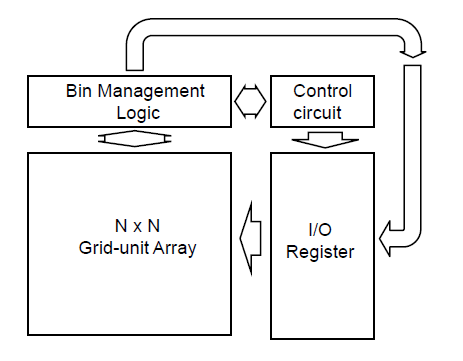The grid block is an array of $\textit{N}$${\times}$$\textit{N}$ grid units. The principal function of the grid unit is a 1${\times}$2 demultiplexer and the array is used to count the number of 1s in a bin. The bin management (BM) block creates bins, deactivates useless grid units, and controls progress of a cycle. The flow of entire sorting process is governed by the control block.

### 2. Operation of The Sorting Grid

The operation of the SG is explained by using a simple example. The SG supports two types of inputs. The one type is key-only inputs where keys themselves are the objects of sorting. In many applications, raw data are the keys to be sorted. The value of key is everything they need so that no other information is necessary. For this type of inputs, stability of sorting is not necessary because there is no way to distinguish two identical data.

The other type of inputs is that key is only a part of input element. Typically, an input element is composed of a key and an object. The key represents a weight of the object. Sorting is performed to determine an order of the objects, although it is done using keys. Various objects can be possible for sorting problems so that a pointer is used to retrieve the object. For this type of inputs, stability is an important property of sorting operation.

For the $\textit{m}$-bit ($\textit{m}$ = $\textit{k}$+$\textit{p}$) inputs with $\textit{k}$-bit key and $\textit{p}$-bit pointer, the I/O block requires ($\textit{m}$+1)-bit shift registers which can be divided by three fields (key, TB, and pointer). Keys must have a fixed size. One bit called tie-break (TB) bit is inserted (with 0) between key field and pointer field. This bit is used to achieve stable sort when multiple inputs have the same key. This bit is inserted/removed by the control block when data is transmitted between I/O block and external systems. For key-only inputs, TB-bit is not necessary, so that $\textit{m}$(=$\textit{k}$)-bit register is sufficient for sorting. The value of $\textit{k}$, and $\textit{p}$ must be given to the control block before input transmission.

##### Fig. 4. Block diagram of the bin-management unit.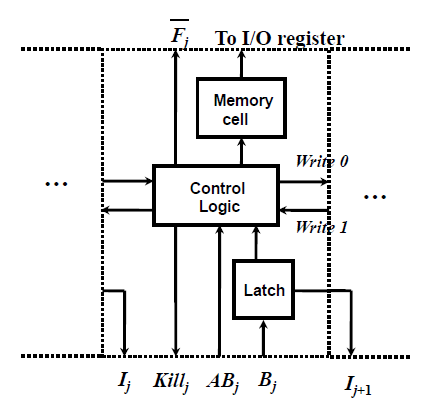The flow of entire sorting process is governed by the control block. The control block generates various asynchronous signals as soon as possible for high-speed operation. The entire sorting operation is performed in $\textit{m}$+1 cycles but each cycle is performed with different latencies. A small bin can be processed more quickly than a large bin. The number of bins increases while the sizes of bins decrease along the cycle. Because all bins are sorted in parallel, the processing time for a cycle keeps decreasing. For this reason, the SG avoids using the fixed period clock. Instead, it uses a self-timed asynchronous clock which flips as soon as possible by checking the states of BM-units. However, it uses a fixed external clock when the I/O block sends/receives data to/from external systems.

Note that three main blocks, the grid array, the I/O block, and the BM-block, have a modular structure. Each block is made by copying a basic unit. Therefore, the engine could be easily synthesized if hardware IP for the grid unit and the BM-unit are available since shift register is included already as a standard cell for most of ASIC tools. The control block can also be easily synthesized by using the conventional control logic design method.

### 4. Performance Enhancement Scheme

Two speed enhancement schemes are developed to enhance the performance of the SG. A self-timed asynchronous clock is used to avoid the inefficiency of the fixed period clock, and a bypassing scheme is employed to decrease the delay of ball propagation time in the grid block.

$\textit{N}$ of $\textit{m}$-bit inputs with $\textit{k}$-bit key and $\textit{p}$-bit pointer are sorted in ($\textit{k}$+$\textit{p}$+1) cycles with the SG. If a fixed period clock is used in the operation, the period of the clock, $\textit{T}$$_{clk}, should be decided as the longest cycle among (\textit{k}+\textit{p}+1) cycles, and the total delay of sorting (\textit{T}$$_{d}$) becomes ($\textit{m}$+1)$\textit{T}$$_{clk}. Since the longest cycle time is proportional to \textit{N}, time complexity of the proposed engine would be \textit{O}(\textit{mN}) when the fixed period clock is used. However, the execution time of the longest cycle could be tens of times longer than that of the shortest cycle, so that fixed period clock is quite inefficient in performance. The execution time of a cycle (\textit{T}$$_{cycle}$) can be analyzed with 4 components.

##### (1)
T c y c l e = T c o l + T B M + T C + T R

where $\textit{T}$$_{col} is the time that a ball propagates from the bottom to the top of the array, and \textit{T}$$_{BM}$ is the time for setting BM-units from the ball position to the boarders of the bin. $\textit{T}$$_{C} is the time spent in the control block and \textit{T}$$_{R}$ is restoring time for the next cycle. The contributions of $\textit{T}$$_{C}and \textit{T}$$_{R}$ to total sorting time are small, and their variations along the cycles are negligible compared to the other two terms, so that they can be regarded as constants. $\textit{T}$$_{col} is proportional to the number of grid units in a column and \textit{T}$$_{BM}$ is proportional to bin size, i.e. the number of columns in a bin. The total delay of sorting $\textit{T}$$_{d} is ##### (2) T d = j = 1 m + 1 T c y c l e , j = j = 1 m + 1 ( T c o l + T B M + α ) j = m + 1 N t g + j = 1 m + 1 ( b max j t B ) j + m + 1 α where, \textit{t}$$_{g}$ is the delay of a grid unit, $\textit{b}$$_{\mathrm{max}} is the largest bin size in cycle \textit{j}, \textit{t}$$_{B}$ is the delay of a BM-unit, and ${\alpha}$ = $\textit{T}$$_{C}+ \textit{T}$$_{R}$ is a constant.

The longest $\textit{T}$$_{cycle} occurs in the first cycle because the bin size is biggest at the first cycle. Although the number of bins increases along the cycle, the size of each bin decreases. Since all bins are processed simultaneously, \textit{T}$$_{BM}$ is determined by the biggest bin in that cycle. Because $\textit{b}$$_{\mathrm{max}} decreases monotonically, \textit{T}$$_{cycle}$ decreases along the cycle, and all bins have only one element after the tie-break ($\textit{k}$+1) cycle. Therefore, the worst-case cycle time at the first cycle is

##### (3)
T c y c l e ,1 s t = N t g + N t B + α

while the shortest cycle time in transmission cycles is

##### (4)
T c y c l e , t r a n s = N t g + t B + α

Eqs. (3) and (4) show a large difference between the longest and shortest cycle times. By reflecting this result, the new sorting engine uses a self-timed asynchronous clock to increase operation speed. The clock flips by detecting the end of critical processes so that each cycle starts as soon as possible. By employing the variable period clock, the time complexity of $\textit{T}$$_{BM} can be reduced from \textit{O}(\textit{Nm}) to \textit{O}(\textit{N+m}) in the best case. Assume that inputs are well balanced so that balls bisect bins in every cycle, then \textit{b}$$_{\mathrm{max,j}}$ would be $\textit{N}$/2$^{j}$. The $\textit{b}$$_{\mathrm{max,j}} decreases until it becomes 1, and rest of the cycles are working with the same \textit{T}$$_{BM}$. If $\textit{N}$ < 2$^{k}$, approximately,

##### (5)
\begin{aligned} \sum T_{B M} &=\sum_{j=1}^{m+1}\left(b_{\max j} t_B\right)_j \\ &=\sum_{j=1}^{k+1}\left(b_{\max j} t_B\right)_j+p t_B \\ &=N\left(\frac{1}{2}+\frac{1}{4}+\ldots+\frac{1}{2^{k+1}}\right) t_B+p t_B \\ & \approx(N+p) t_B \end{aligned}

which has $\textit{O}$($\textit{N+m}$) time complexity. The distribution of pseudo-random inputs shows similar patterns as the best case.

Unlike $\textit{T}$$_{BM}, \textit{T}$$_{col}$ is not changed even though the number of active grid units in a column keeps decreasing. The columns in a bin are localized in the array, but the active grid units are scattered over entire column. Even if most of units in a column are disabled, a ball should pass those units so that a ball must pass $\textit{N}$ grid units regardless of the number of inactive units. Although $\textit{T}$$_{BM} is much larger than \textit{T}$$_{col}$ in early cycles, $\textit{T}$$_{col} becomes the dominant component from few cycles later causing \textit{T}$$_{d}$ to remain in $\textit{O}$($\textit{Nm}$).

To resolve this problem, a bypass scheme is developed as in Fig. 5. The purpose of the bypass scheme is to skip consecutive inactive grid units in ball-propagation. A column is divided into several groups such that every group consists of consecutive $\textit{q}$ grid units and has a bypassing line. If all grid units in a group are disabled, a ball does not pass the grid units in the group, but the ball is sent to the next group through the bypassing line. The bypassing scheme is very effective in later cycles since lots of grid units are disabled.

##### Fig. 5. Basic grid block with the first level bypassing circuit.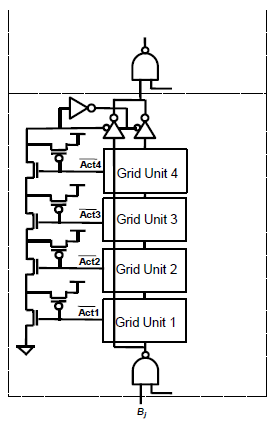Fig. 5 shows the basic block of grid units and implementation of the first level bypassing scheme. Four grid units with a repeater form a basic grid-block which is used as a basic module of ASIC library. With the first level bypassing scheme, only 4 times is the maximum achievable speed-up. For greater speed up, multi-level bypassing scheme could be employed. The number of levels and the number of grid units in a group should be decided considering the applications and hardware overheads.

Similarly, with $\textit{T}$$_{BM}, time complexity of \textit{T}$$_{col}$ can be $\textit{O}$($\textit{N+m}$) in the best case with an optimal input distribution and the maximal level bypassing scheme. Therefore, the overall latency ($\textit{T}$$_{d}) of the sorting engine can achieve \textit{O}(\textit{N+m}) time-complexity, but \textit{O}(\textit{Nm}) in the worst-case distribution. ## IV. PERFORMANCE EVALUATION The performance of SG is evaluated through simulations with pseudo-random inputs. The simulation is performed by HSPICE with 1.2 V 0.13 {\mu}m-process model parameter. Simulations are performed with several input sizes and key sizes. For each type of inputs, 10 samples are simulated, and their average values are used as the results. Two groups of inputs are used in simulation where one group uses 8-bit key and the other does 16-bit key. The inputs with 16, 64, 256, and 1024 elements are used. Key-only inputs are used for simulations because transmission cycles are just moving data with a constant cycle time. Multi-level bypassing scheme is used such that every 4 block is grouped as a bypassing unit. For example, 4-level (maximum level) bypassing scheme is used in sorting 1024 inputs. Table 1 shows the simulation results. The proposed sorting engine sorts 8-bit 1024 inputs in 1.042 {\mu}s. Its rate is about one element per nano-second. Even when the key size is increased twice, the sorting time increases by 55% for 16 inputs while it increases by 29% only for 256 inputs. For 1024 inputs, the sorting time increases only by 6%. One reason of such an amazing result is that 1024 inputs with 8-bit key include too many identical inputs since \textit{N} > 2^{k}, while \textit{N} < 2^{k} for 16-bit key inputs. The efficiency of bypassing scheme drops when inputs include many same-key inputs. As in Table 1, the gap in cycle times between the first cycle and the last cycle is very large. The difference is getting bigger for a larger number of inputs. Fig. 6 shows how the cycle time (\textit{T}$$_{cycle}$) changes along the cycles. $\textit{T}$$_{cycle} decreases rapidly along the cycle and is saturated at a point. It is because \textit{T}$$_{col}$ and $\textit{T}$$_{BM} keep decreasing but \textit{T}$$_{C}$+$\textit{T}$$_{R} does not. Therefore, \textit{T}$$_{C}$+$\textit{T}$$_{R} becomes the dominant factor of \textit{T}$$_{cycle}$ from the point.

##### Table 1. Simulation Results
 # of Input 8-bit Key 16-bit Key $T_{d}$ (ns) $T_{cycle}$ (ns) $T_{d}$ (ns) $T_{cycle}$ (ns) First Last First Last 16 25.3 5.7 2.0 39.3 5.4 1.9 64 69.3 19.9 3.6 100.0 20.3 3.2 256 253.3 79.6 6.8 325.6 80.8 4.9 1024 1042.2 318.4 26.2 1105.1 317.0 7.1

If $\textit{N}$ > 2$^{k}$, there must be multiple inputs with a same key as the case of 1024 inputs with 8-bit. This input may have 4 elements for any key value so that the efficiency of the bypassing scheme is reduced. It can be detected in Fig. 6(a) that $\textit{T}$$_{cycle} of 1024 inputs decreases slowly than that of 256 inputs where only few inputs may be the same. It implies that the bypassing scheme is more efficient when \textit{N} < 2^{k}. ##### Fig. 6. Change of cycle time: (a) inputs with 8-bit key; (b) inputs with 16-bit key.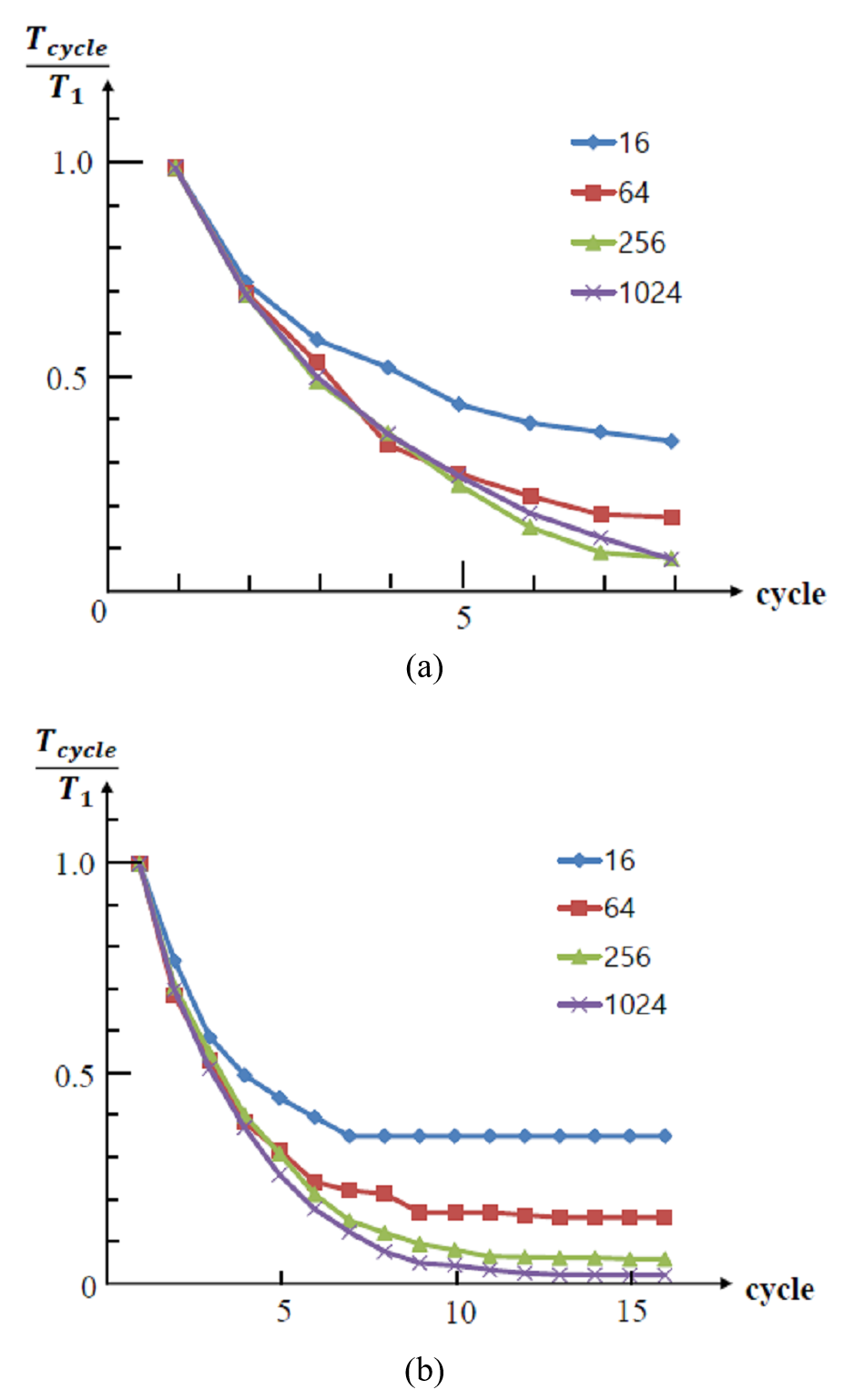The shortest cycle time is about 44 times faster than the longest cycle for 1024 inputs with 16-bit key. If a fixed period clock is used, the sorting delay (\textit{T}$$_{d}$) would be 2.25 ${\mu}$s (16${\times}$317 ns) which is double the delay of SG (1.105 ${\mu}$s). The largest difference occurs when sorting 256 inputs with 16-key, which the delay is quadrupled. It proves that employing the variable period clock makes the engine more than two times faster than using a fixed cycle clock.

The speed of SG is compared to that of other comparison-free sorting engines. The comparison is summarized in Table 2. Only the sorting engine in  is implemented using FPGA while the others are done with VLSI design, thus it has difficulties in direct comparison with other engines. The number of cycles required to complete sorting operation is $\textit{k}$+1 for SG which is fixed regardless of the number of inputs ($\textit{N}$), while it is proportional to $\textit{N}$ for the other sorting engines. The total sorting time ($\textit{T}$$_{D}) of SG is only affected by clock cycle time (\textit{T}$$_{cycle}$) so that SG uses the self-timed asynchronous clock to increase the sorting speed.

##### Table 2. Comparison of SG with other sorting engines
 SG in  in  in  in  Time complexity O(N+k) O(N+k) O(N+2k) O(N) O(N) Restriction None None N > k N${\approx}$2$^{k}$ None Technology 130 nm 350 nm 65 nm 90 nm FPGA (65 nm) N 1024 63 16 1024 1024 k 16 16 16 10 16 No. of cycles 17 78 48 2k~3k 1024 ( k+1 ) ( N+k-1 ) ( N+2k ) (2N~3N) (N~2N) T$_{\mathrm{cycle}}$ variable 5 ns 1.6 ns 2 ns 7.8 ns Sorting Time (T$_{D}$) 1105.1 ns 390 ns 76.8 ns 4~6 ms 8 ms T$_{D}$ / N 1.08 ns 6.19 ns 4.8 ns 4~6 ns 7.8 ns

Because the sorting engines use different key sizes and data sizes in speed simulation, the sorting time per element ($\textit{T}$$_{D}/\textit{N}) is used to compare their speeds. As in Table 2, SG is the fastest among all comparison-free sorting engines. The sorting rate of SG is about 1 element per 1 ns while those of other engines are 0.13 ~ 0.25 element per 1 ns, where SG is more than 4 times faster than other engines. ## V. CONCLUSIONS A novel sorting engine called Sorting Grid is presented in this paper. It implements radix-2 sorting algorithms with VLSI design. Although SG has \textit{O}(\textit{N}$$^{2}$) hardware complexity due to the $\textit{N}$${\times}$$\textit{N}$ grid array, it uses minimal memory space in sorting so that its hardware requirement is less than CAS-based sorting engines. SG sorts $\textit{m-}$bit $\textit{N}$ binary inputs in ($\textit{m}$+1) cycles with variable cycle times. The cycle times of SG decrease greatly along the cycle so that self-timed asynchronous clock with variable period is employed. By the clock and multi-level bypassing scheme, SG can achieve $\textit{O}$($\textit{N+m}$) time-complexity for pseudo-random binary inputs. The speed of SG is evaluated by simulations. The results show that the sorting rate of SG is about 1 ns/input for 16-bit binary inputs.

SG is intended to be used as a hardware module for ASIC design which can be employed for any ASIC chip requiring fast sorting operations. The engine has simple modular architecture so that it is easily synthesized with conventional ASIC tools if hardware IPs for the grid unit and the BM-unit are prepared.

## References

1
Najafi M. H., Lilja D. J., Riedel M. D., Bazargan K., 2018, Low-cost sorting network circuits using unary processing, IEEE Transactions on Very Large Scale Integration (VLSI) Systems, Vol. 26, No. 8, pp. 1471-14802
Li P., Lilja D. J., Qian W., Bazargan K., Riedel M. D., 2014, Computation on stochastic bit streams digital image processing case studies, IEEE Transactions on Very Large Scale Integration (VLSI) Systems, Vol. 22, No. 3, pp. 449-4623
Graefe G., Sep. 2006., Implementing sorting in database systems, ACM Computing Surveys, Vol. 38, No. 3, pp. 104
Chang R. C., Wei M., Chen H., Lin K., Chen H., Gao Y., Lin S., 2014, Implementation of a high-throughput modified merge sort in mimo detection systems, IEEE Transactions on Circuits and Systems I, Vol. 61, No. 9, pp. 2730-27375
Colavita A. A., Cicuttin A., Fratnik F., Capello G., 2003, Sortchip: a vlsi implementation of a hardware algorithm for continuous data sorting, IEEE Journal of Solid-State Circuits, Vol. 38, No. 6, pp. 1076-10796
Tang Y., Bergmann N. W., 2015, A hardware scheduler based on task queues for fpga-based embedded real-time systems, IEEE Transactions on Computers, Vol. 64, No. 5, pp. 1254-12677
KohÃžtka L., StopjakovÃa˛ V., 2017, Rocket queue: New data sorting architecture for real-time systems, IEEE 20th International Symposium on Design and Diagnostics of Electronic Circuits Systems (DDECS), pp. 207-2128
Yan J. T., 1999, An improved optimal algorithm for bubble-sorting-based non-Manhattan channel routing, IEEE Trans. Comput.-Aided Des. Integr. Circuits Syst., Vol. 18, No. 2, pp. 163-1719
Campobello G., Russo M., Oct. 2006, A scalable VLSI speed/area tunable sorting network, J. Syst. Archit., Vol. 52, No. 10, pp. 589-60210
Tabrizi N., Bagherzadeh N., Oct. 2005, An ASIC design of a novel pipelined and parallel sorting accelerator for a multiprocessor-on-a-chip, in Proc. IEEE 6th Int. Conf. ASIC (ASICON), pp. 46-4911
Demirci T., Hatirnaz I., Leblebici Y., 2003, Full-custom CMOS realization of a high-performance binary sorting engine with linear area-time complexity, in International Symposium on Circuits and Signals, pp. 453-45612
Alaparthi S., Gulati K., Khatri S. P., 2009, Sorting binary numbers in hardware - A novel algorithm and its implementation, in International Symposium on Circuits and Systems, pp. 2225-222813
Abdel-Hafeez S., Gordon-Ross A., 2017, An efficient o(n) comparisonfree sorting algorithm, IEEE Transactions on Very Large Scale Integration (VLSI) Systems, Vol. 25, No. 6, pp. 1930-194214
Ray S. S., Adak D., Ghoch S., Worst-Case O(N) comparison-free Hardware Sorting Engine, IEEE Transactions on Computer-Aided Design of Integrated Circuits and Systems, 2022, to be published15
Wikipedia, https://en.wikipedia.org/wiki/Sorting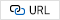Myungchul Yoon received the BS and MS degrees in Electronics Engineering from Seoul National University, Korea, in 1986 and in 1988 respectively, and the Ph.D. degree in Electrical and Computer Engineering from the University of Texas at Austin in 1998. From 1988 to 2002, he was with Hynix Inc. Icheon, Korea as technical research staff at Semiconductor R&D Lab., and Mobile Communication R&D Lab. From 2005 to 2006, he was with DGIST, Korea as technical staff at the Information Technology R&D Division. Since 2006, he has been with the Department of Electronics and Electrical Engineering, Dankook University, YongIn, Korea, where he is a professor. His research interests are in low-power VLSI design, embedded systems, and mobile communication.